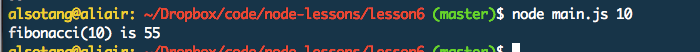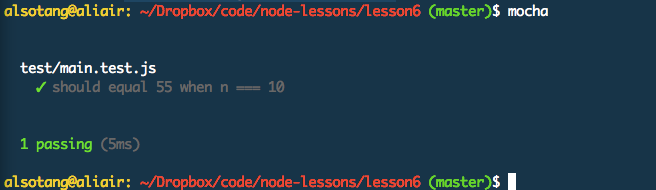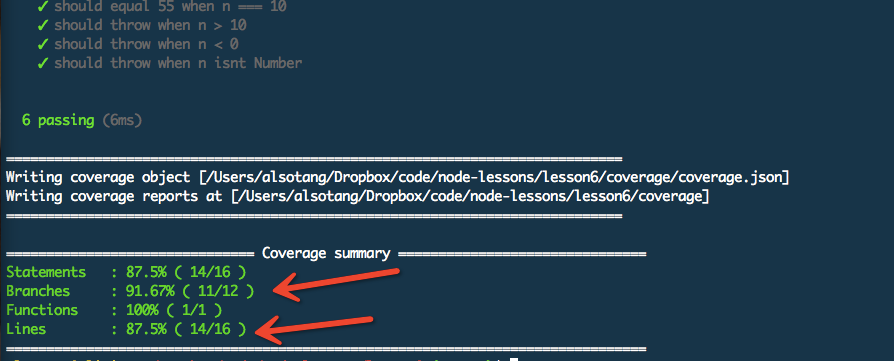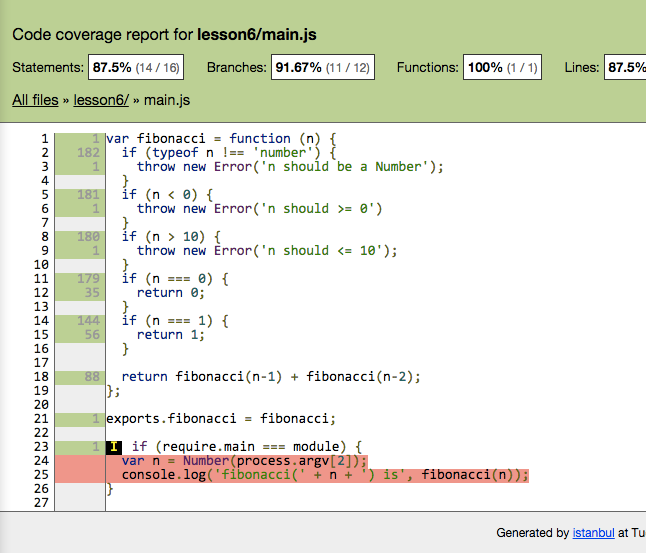# mocha, should, istanbul

### 《测试用例：mocha，should，istanbul》

#### 目标

main.js: 其中有个 fibonacci 函数。fibonacci 的介绍见：http://en.wikipedia.org/wiki/Fibonacci_number

• 当 n === 0 时，返回 0；n === 1时，返回 1;
• n > 1 时，返回 `fibonacci(n) === fibonacci(n-1) + fibonacci(n-2)`，如 `fibonacci(10) === 55`;
• n 不可大于10，否则抛错，因为 Node.js 的计算性能没那么强。
• n 也不可小于 0，否则抛错，因为没意义。
• n 不为数字时，抛错。

test/main.test.js: 对 main 函数进行测试，并使行覆盖率和分支覆盖率都达到 100%。

#### 知识点

1. 学习使用测试框架 mocha : http://mochajs.org/
2. 学习使用断言库 should : https://github.com/tj/should.js
3. 学习使用测试率覆盖工具 istanbul : https://github.com/gotwarlost/istanbul
4. 简单 Makefile 的编写 : http://blog.csdn.net/haoel/article/details/2886

#### 课程内容

``````var fibonacci = function (n) {
if (n === 0) {
return 0;
}
if (n === 1) {
return 1;
}
return fibonacci(n-1) + fibonacci(n-2);
};

if (require.main === module) {
// 如果是直接执行 main.js，则进入此处
// 如果 main.js 被其他文件 require，则此处不会执行。
var n = Number(process.argv);
console.log('fibonacci(' + n + ') is', fibonacci(n));
}
``````

OK，这只是个简单的实现。

`\$ node main.js 10``exports.fibonacci = fibonacci;`（要是看不懂这句就去补补 Node.js 的基础知识吧）

``````// file: test/main.test.js
var main = require('../main');
var should = require('should');

describe('test/main.test.js', function () {
it('should equal 55 when n === 10', function () {
main.fibonacci(10).should.equal(55);
});
});
``````

`-g` 与 非`-g` 的区别，就是安装位置的区别，g 是 global 的意思。如果不加的话，则安装 mocha 在你的项目目录下面；如果加了，则这个 mocha 是安装在全局的，如果 mocha 有可执行命令的话，那么这个命令也会自动加入到你系统 \$PATH 中的某个地方（在我的系统中，是这里 `/Users/alsotang/.nvm/v0.10.29/bin`

`\$ mocha`https://github.com/cnodejs/nodeclub/blob/master/test/controllers/topic.test.js

`describe` 中的字符串，用来描述你要测的主体是什么；`it` 当中，描述具体的 case 内容。

should 在 js 的 Object “基类”上注入了一个 `#should` 属性，这个属性中，又有着许许多多的属性可以被访问。

should.js 如果现在还是 version 3 的话，我倒是推荐大家去看看它的 API 和 源码；现在 should 是 version 4 了，API 丑得很，但为了不掉队，我还是一直用着它。我觉得 expect 麻烦，所以不用 expect，对了，expect 也是一个断言库：https://github.com/LearnBoost/expect.js/ 。

• 当 n === 0 时，返回 0；n === 1时，返回 1;
• n > 1 时，返回 `fibonacci(n) === fibonacci(n-1) + fibonacci(n-2)`，如 `fibonacci(10) === 55`;
• n 不可大于10，否则抛错，因为 Node.js 的计算性能没那么强。
• n 也不可小于 0，否则抛错，因为没意义。
• n 不为数字时，抛错。

``````var main = require('../main');
var should = require('should');

describe('test/main.test.js', function () {
it('should equal 0 when n === 0', function () {
main.fibonacci(0).should.equal(0);
});

it('should equal 1 when n === 1', function () {
main.fibonacci(1).should.equal(1);
});

it('should equal 55 when n === 10', function () {
main.fibonacci(10).should.equal(55);
});

it('should throw when n > 10', function () {
(function () {
main.fibonacci(11);
}).should.throw('n should <= 10');
});

it('should throw when n < 0', function () {
(function () {
main.fibonacci(-1);
}).should.throw('n should >= 0');
});

it('should throw when n isnt Number', function () {
(function () {
main.fibonacci('呵呵');
}).should.throw('n should be a Number');
});
});
``````

``````var fibonacci = function (n) {
if (typeof n !== 'number') {
throw new Error('n should be a Number');
}
if (n < 0) {
throw new Error('n should >= 0')
}
if (n > 10) {
throw new Error('n should <= 10');
}
if (n === 0) {
return 0;
}
if (n === 1) {
return 1;
}

return fibonacci(n-1) + fibonacci(n-2);
};
``````mocha 和 istanbul 的结合是相当无缝的，只要 mocha 跑得动，那么 istanbul 就接得进来。

1. `\$ npm i mocha --save-dev`，装个 mocha 到项目目录中去
2. `\$ ./node_modules/.bin/mocha`，用刚才安装的这个特定版本的 mocha，来跑项目的测试代码。

`./node_modules/.bin` 这个目录下放着我们所有依赖自带的那些可执行文件。

``````test:
./node_modules/.bin/mocha

cov test-cov:
./node_modules/.bin/istanbul cover _mocha

.PHONY: test cov test-cov
``````